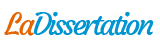# Formulas class

Cours : Formulas class. Recherche parmi 240 000+ dissertations

Par   •  12 Mars 2019  •  Cours  •  1 452 Mots (6 Pages)  •  89 Vues

Page 1 sur 6

Formulas

Future Value and Present Value:

Future value of uneven or irregular cash flows:

Present value of uneven or irregular cash flows:

Special cases:

Future value interest factor (with \$1 at 0):

Present value interest factor (with \$1 at time n):

For Ordinary annuity, i.e., the first \$1 at time 1 and the last \$1 at time n,

Future value interest factor of annuity, denoted as :

Present value of interest factor of annuity, denoted as :

For Annuity due, i.e., the first \$1 at time 0 and the last \$1 at time n-1,

FV of annuity due = (1+r) ×FVIFA(r, n)

PV of annuity due = (1+r) ×PVIFA (r, n)

Present value of perpetuity: .

Amortized loan:

Payment of amortized loan:

Mortgage Balance at the end of period t: .

Interest portion of the (t+1)th mortgage payment =

Principal portion of the (t+1)th mortgage payment = PMT – Interest portion

Interest rate quote and conversion:

The quoted annual rate is called the nominal rate or the annual percentage rate (APR), which must be first converted to the effective annual rate (EAR) before seeking for the interest rate per period as required by the application.

The nominal rate is often quoted as annual percentage rate (APR), which is defined as APR = m×rperiod. It is often referred to as the simple interest rate, because the interests earned are added, not compounded. It is legal if and only if the quoted rate clearly indicates its compounding assumption. If an APR quote indicates semiannual compounding, m = 2. Denote rperiod = rs. This quote can only be interpreted as . If it indicates monthly compounding, m = 12. Denote rperiod = rmonth. In this case, the quote can only be interpreted as . The APR can be converted to the EAR (effective annual rate) by the relationship:

.

This relationship is valid if and only if the m is consistent with the compounding assumption of the APR quote.

Denote as the interest rate per period of the application which requires k times compounding per year. This rate can be found by taking the kth root:

.

For example, the semiannual rate is ;

The monthly rate is .

The can be solved directly from the APR quote as

.

In a special case where the m value of the compounding assumption coincides with the compounding requirement of the application, i.e., k = m, the above is simplified to

.

This formula is valid if and only if k = m.

Canadian Mortgage Rate Calculation: The nominal mortgage rate is quoted as APR with semiannual compounding. To find the effective interest rate per month, denoted as , first find

, then . It can also be found in one step as

.

Bond Pricing and Yield

Denote F as the par or face value of the bond, i as the annual coupon rate, N as the maturity (in years). The annual coupon interest payment is calculated as .

If the bond pays annual coupon interest (i.e., once a year), the price or value of the bond is the present value of the future cash flows:

,

where is called the yield to maturity (YTM) or just the yield, which is quoted as the EAR. If the bond price is known (e.g., observed), the YTM is a trial value that satisfies the above equation.

Denote as a trial value on the yield. Find a trial price by the above formula as

.

If the pricing error is within an acceptable range, i.e.,

,

then can be accepted as the yield or the YTM. The trial value can be approximated as

If the bond is callable in years from now ( ), the Yield to Call (YTC) is defined as a trial value such that

,

where is the calling price. The YTC can also be approximated as

The pricing error is defined the same way as the above.

Because the North American bonds pay coupon interests semiannually, the bond yield or YTM, denoted as y, is quoted as APR with the semiannual compounding assumption. The standard bond pricing formula for semiannual payment bond is

,

where , rs = y/2, and n=2N.

If the bond price P is known or observed, the yield to maturity (YTM) can be found by trial and error on such that the above equation is satisfied. The YTM is reported as , which is an APR quote based on the semiannual compounding assumption. The trial value can be approximated as

, then .

If the bond is callable, the YTC is defined as such that

...

Télécharger au format  txt (7.6 Kb)   pdf (44.9 Kb)   docx (12.4 Kb)
Voir 5 pages de plus »